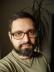## Forward difference series

Create a script that prints nth order forward difference series. You should be a able to pass the list of numbers and order number as command line parameters.
Series592816
Order
1(9-5)(2-9)(8-2)(1-8)(6-1)
4-76-75
2(-7-4)(6+7)(-7-6)(5+7)
-1113-1312
3(13+11)(-13-13)(12+13)
24-2625
4(-26-24)(25+26)
-5051
5(51+50)
101

I started with a simple idea: Let’s just subtract the neighbouring elements and populate a new sequence. If the order is greater than 1, recursively call the same function.

``````sub fds {
my (\$seq, \$order) = @_;
my @r = map \$seq->[\$_] - \$seq->[\$_ - 1], 1 .. \$#\$seq;
return @r if \$order == 1;

return fds(\@r, \$order - 1)
}``````

And it passes the tests based on the example above:

``````use Test::More tests => 5;

my \$example = [5, 9, 2, 8, 1, 6];
is_deeply [fds(\$example, 1)], [4, -7, 6, -7, 5];
is_deeply [fds(\$example, 2)], [-11, 13, -13, 12];
is_deeply [fds(\$example, 3)], [24, -26, 25];
is_deeply [fds(\$example, 4)], [-50, 51];
is_deeply [fds(\$example, 5)], ;``````

“Is there a way to solve it without recursion?” I asked myself. I replaced the numbers in the example table with symbols and searched for a pattern:

```fds(1) = xi+1 - xi
fds(2) = xi+2 - 2xi+1 + xi
fds(3) = xi+3 - 3xi+2 + 3xi+1 - xi
fds(4) = xi+4 - 4xi+3 + 6xi+2 - 4xi+1 + xi
...```

Do you see it yourself? The coefficients correspond to Pascal’s (or Tartaglia’s) triangle, while they signs periodically change.

To get the binomial coefficient, we need to compute the nCk. The formula is simple:

`nCk = n! / k! / (n-k)!`

In Perl, it can be written as

``````sub binomial {
my (\$n, \$k) = @_;
my \$r = 1;
\$r *= \$_ for 2 .. \$n;
\$r /= \$_ for 2 .. \$k, 2 .. (\$n - \$k);
return \$r
}``````

As the computation involves several loops (all the factorials), let’s cache the results to improve the speed:

``````{   my %cache;
sub binomial {
my (\$n, \$k) = @_;
return \$cache{\$n}{\$k} if \$cache{\$n}{\$k};
my \$r = 1;
\$r *= \$_ for 2 .. \$n;
\$r /= \$_ for 2 .. \$k, 2 .. (\$n - \$k);
return \$cache{\$n}{\$k} = \$r
}
}``````

The forward difference series just needs to compute the coefficients, change their sign if needed, and sum the sequences.

``````use List::Util qw{ sum };

sub fds2 {
my (\$seq, \$order) = @_;
my @coefficients = map binomial(\$order, \$_), 0 .. \$order;
\$coefficients[2 * \$_] *= -1 for -(\$order + 1) / 2 .. -1;
return map {
my \$i = \$_;
sum(map \$seq->[\$i + \$_] * \$coefficients[\$_], 0 .. \$order)
} 0 .. \$#\$seq - \$order
}``````

The higher the order, the faster the second approach becomes compared to the original one. But once the order gets too great (about 40 for a sequence `map int rand 100, 1 .. 50`) the results become wrong: the product of `2 .. \$n` becomes too large for an integer, so Perl turns it into a float, introducing subtle errors of imprecision.

I tried to fix that, but I needed a solution to the second task first.

## Prime decomposition

Create a script that prints the prime decomposition of a given number. The prime decomposition of a number is defined as a list of prime numbers which when all multiplied together, are equal to that number. For example, the prime decomposition of 228 is 2,2,3,19 as 228 = 2 * 2 * 3 * 19.

When searching for the primes that divide the given number \$n, we have to check all the primes from 2 to \$n to find those that divide \$n. In fact, we can check all the numbers \$i in the range: first check whether we have a prime, and if so, we can check whether it divides \$n. When checking whether \$i is a prime, we already know all the primes less than \$i, so we can easily check whether any of them divides \$i.

``````use List::Util qw{ any };

sub prime_decomposition {
my (\$n) = @_;
my %primes;
for my \$i (2 .. \$n) {
next if any { 0 == \$i % \$_ } keys %primes;

\$primes{\$i} = 0;
until (\$n % \$i) {
++\$primes{\$i};
\$n /= \$i;
}
last if 1 == \$n;
}
return map +(\$_) x \$primes{\$_}, sort { \$a <=> \$b } keys %primes
}``````

`\$primes{\$i}` says how many times the prime \$i occurs in the prime decomposition of \$n.

## Back to forward difference series

Now, we don’t need to calculate the factorials. When calculating the binomial coefficient, we can find the prime decomposition of both the numerator and denominator and cancel all the common primes out.

Note that we don’t need to sort the returned primes which gives us a negligible performance improvement.

``````use List::Util qw{ product };

{   my %cache;
sub binomial {
my (\$n, \$k) = @_;
return \$cache{\$n}{\$k} if \$cache{\$n}{\$k};

my %numerators;
++\$numerators{\$_}
for map prime_decomposition(\$_), \$k + 1 .. \$n;
delete \$numerators{1};
my %denominators;
++\$denominators{\$_}
for map prime_decomposition(\$_), 2 .. \$n - \$k;

DENOM:
while (keys %denominators) {
for my \$d (keys %denominators) {
for my \$u (keys %numerators) {
if (0 == \$u % \$d) {
--\$numerators{\$u} or delete \$numerators{\$u};
--\$denominators{\$d} or delete \$denominators{\$d};
my \$new = \$u / \$d;
++\$numerators{\$new} unless 1 == \$new;
next DENOM
}
}
}
}

my \$r = product(map +(\$_) x \$numerators{\$_}, keys %numerators)
/ product(keys %denominators);
return \$cache{\$n}{\$k} = \$r
}
}``````

Note that the denominators never occur more than once, so we can skip the mapping needed for numerators in the final products.

Unfortunately, the improvement of the solution isn’t substantial. Its performance is slightly better (cmpthese from Benchmark shows about 15%), but the results don’t stay correct for much larger order. About the order of 57, the binomial coefficient itself becomes too large to fit into an integer, and Perl turns it into a float. I haven't tried to use Math::BigInt, but I guess the performance hit would have been critical.I blog about Perl.